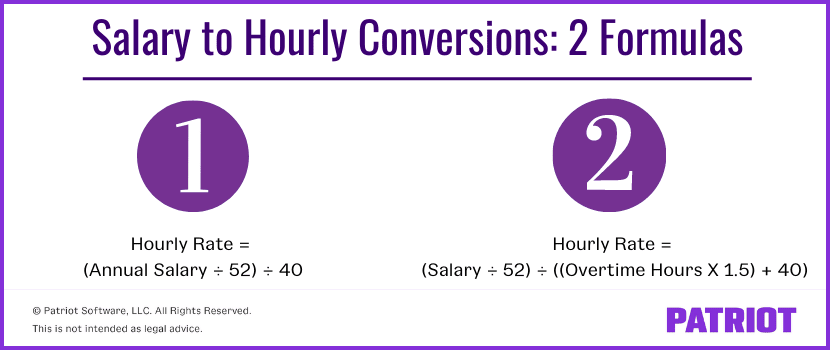How to Convert Salary to Hourly | Formula and Examples

Most popular blog categories

# How to Convert Salary to Hourly for Nonexempt Employees

Sometimes, being an employer feels like you’re doing nonstop math. And if you want to know how to convert salary to hourly rate for employees, it’s time to whip out those calculators.

So, when would you need to convert a salary to hourly wages? You may want to know how an employee’s salary compares to employees who receive hourly wages. Or, you might need to know how to calculate overtime for salaried employees

Regardless of your reason, we’ve got the formulas you need to convert salary to hourly wages below.

## How to convert salary to hourly

Below are two hourly wage calculation methods for salary employees. The first salary to hourly formula is shorter and easier to calculate. The second formula involves more calculations.

However, don’t automatically use the first formula to avoid doing extra math. Both methods come with a downfall, which is explained in each section.### Salary-to-hourly conversion: Method 1

With this salary-to-hourly conversion, you will calculate the hourly rate based on a standard 40-hour workweek. If a standard workweek at your business is less than 40 hours, use that number instead of 40 in the equation.

Here is the basic salary-to-hourly formula for this method:

(Annual salary ÷ 52) ÷ 40 = Hourly Rate

First, divide the employee’s annual salary by 52 weeks (the number of weeks in a year).

\$31,200 ÷ 52 = \$600 per week

Divide the weekly wages by 40 hours.

\$600 ÷ 40 = \$15 per hour

In this example, the employee’s hourly rate is \$15.

#### The downside of method 1

This first method is great to use if the employee does not work much overtime. The method is quick and easy to do. But if the employee does work a lot of overtime, you might pay a lot of overtime wages.

In the example above, the employee’s annual salary was \$31,200 based on a standard 40-hour workweek.

What if you begin paying the employee the \$15 per hour rate, but the employee actually works 45 hours per week?

First, calculate the employee’s earnings for the regular hours they worked during the week.

40 hours × \$15 = \$600 regular weekly earnings

Next, calculate the overtime rate of pay. The overtime rate is one and a half times the regular rate of pay.

\$15 × 1.5 = \$22.50 overtime rate

Multiply the overtime rate of pay by the weekly overtime rate.

\$22.50 × 5 overtime hours = \$112.50 weekly overtime wages

Add together the regular and overtime wages for the week.

\$112.50 + \$600 = \$712.50 total weekly wages

Assuming that the employee consistently works 45 hours per week, you can multiply the total weekly rate by the number of weeks in a year to find the total yearly earnings.

\$712.50 × 52 weeks = \$37,050 total yearly wages

When you use this hourly wage calculation for salary employees, employees who work a lot of overtime will earn more as nonexempt hourly employees than they did when they were exempt salary employees. In this example, the employee would earn \$5,850 more per year.

### Converting salary-to-hourly: Method 2

With the second method, you’ll learn how to convert salary to hourly based on the average hours the employee works each week.

First, you need to know the average number of hours the employee works each week. If you previously had nonexempt salaried employees track their time, use those records to calculate an average.

If you do not have time records, use your best knowledge of how much the employee typically works each week. You can also talk to the employee about how many hours they think they work.

Here is the basic equation for this method:

(Salary ÷ 52) ÷ ((Overtime Hours × 1.5) + 40)  = Hourly Rate

Let’s say your employee earns a salary of \$31,200 per year and works an average of 45 hours per week.

To start, find the employee’s weekly salary. Divide the yearly salary by 52.

\$31,200 ÷ 52 = \$600 weekly salary

Next, figure out how many overtime hours the employee works each week. In this case, the employee works five overtime hours each week (45 – 40 = 5 hours).

Multiply the overtime hours by 1.5. This gives you the number of regular hours that is equivalent to the wages of the overtime hours.

5 × 1.5 = 7.5 hours

7.5 + 40 = 47.5 hours

Divide the employee’s weekly salary by the number of hours.

\$600 ÷ 47.5 = \$12.63 hourly rate

Using this second method, the employee’s hourly wage is \$12.63.

#### The downside of method 2

The second method is good if the employee consistently works overtime hours. But if the employee does not work overtime, they might end up with fewer wages than when they were salaried.

For example, the employee works overtime but ends up only averaging 43 hours per week due to a fluctuating schedule. The employee will have reduced wages.

If this employee only works an average of 43 hours per week they would earn \$29,225.82 per year. That’s \$1,974.18 less per year than they would have earned as a salaried employee. If you use the second method to switch a salaried employee to an hourly employee, you can give a bonus if they do not earn enough to equal their former salary.

Do you want an easy way to calculate employee wages? Try Patriot’s simple Full Service Payroll today! Quickly run payroll with our easy three-step process, and Patriot will handle the taxes for you.

This article has been updated from its original publication date of August 1, 2016.